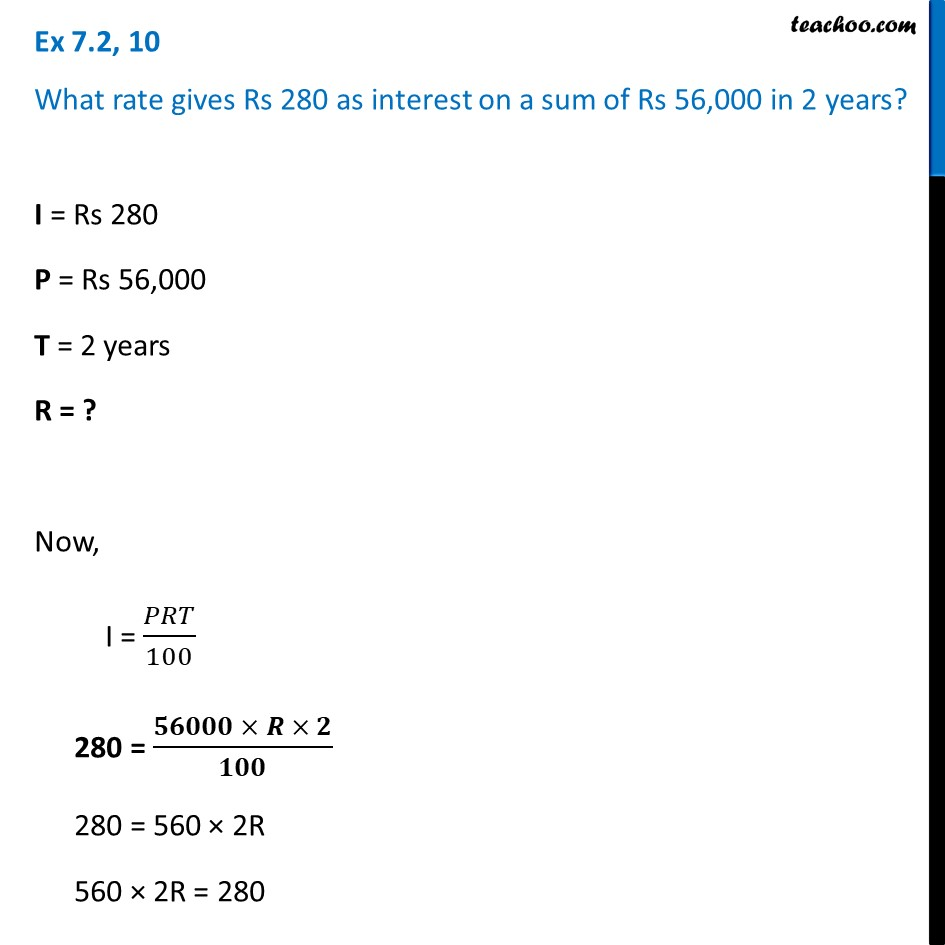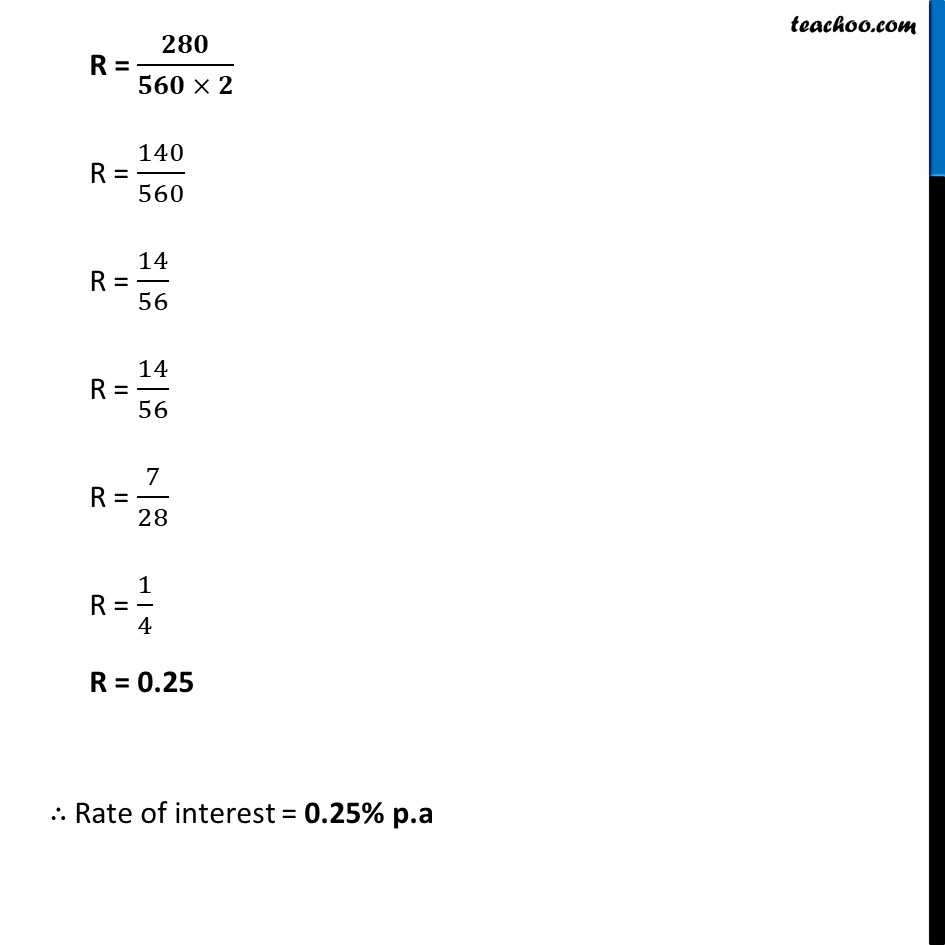Ex 7.2

Chapter 7 Class 7 Comparing Quantities
Serial order wiseLearn in your speed, with individual attention - Teachoo Maths 1-on-1 Class

### Transcript

Ex 7.2, 10 What rate gives Rs 280 as interest on a sum of Rs 56,000 in 2 years? I = Rs 280 P = Rs 56,000 T = 2 years R = ? Now, I = 𝑃𝑅𝑇/100 280 = (𝟓𝟔𝟎𝟎𝟎 × 𝑹 × 𝟐)/𝟏𝟎𝟎 280 = 560 × 2R 560 × 2R = 280 R = 𝟐𝟖𝟎/(𝟓𝟔𝟎 × 𝟐) R = 140/560 R = 14/56 R = 14/56 R = 7/28 R = 1/4 R = 0.25 ∴ Rate of interest = 0.25% p.a